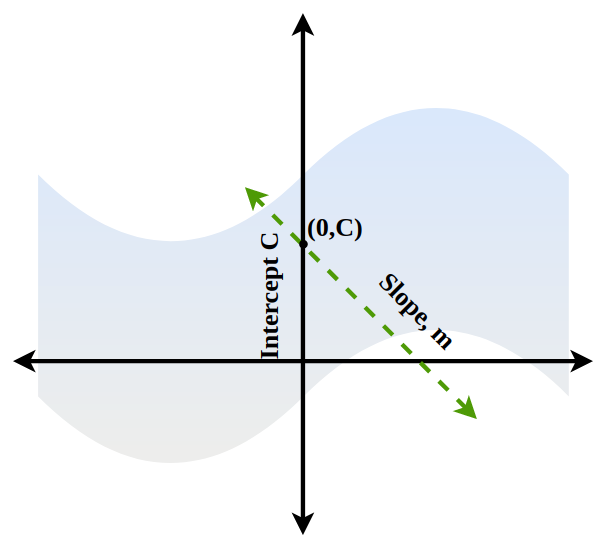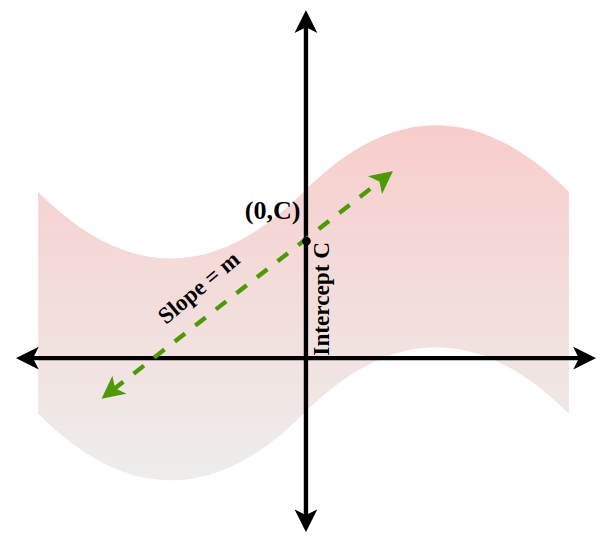Open In App
Related Articles
• CBSE Class 11 Maths Notes
• CBSE Class 11 Maths Formulas
• NCERT Solutions for Class 11 Maths
• RD Sharma Class 11 Solutions for Maths

# Slope Intercept Form

Equation of line is the algebraic way to represent a line. We can represent the equation of a line in various forms. The various ways of representing the equation of a straight line are,

• Slope intercept form
• Intercept form
• Point slope form
• Two point form

Slope intercept form of the equation is used to represent the equation of a straight line when the slope of the equation and the intercept on the y-axis is given.

In this article, we will learn about the slope-intercept form of a straight line, its derivation, graphs, and examples.

## What is Slope Intercept Form of a Line?

Slope Intercept Form of a Line is a way of representing the straight line in the cartesian plane. As the name suggests, in this form we are given the slope of the straight line and its intercept on the y-axis. The general method of representing the straight line in slope intercept form is y = mx + c, where m is the slope of the straight line and c is the intercept of the line on the y-axis.

The slope (m) of the line represents the gradient of the line and is calculated using the slope formula,

m = tanθ

where θ is the angle made by the straight line with the x-axis.

And c is the intercept made by the straight line on the y-axis. If the intercept made by the straight line is in the negative y-axis then the equation of the straight line is, y = mx – c.

## Slope Intercept Form Equation

The slope-intercept form of the equation represents the equation of the straight line having a specific slope and making an intercept with the y-axis.

The slope of the line is the gradient of the line with the x-axis. If the line makes an angle θ then its slope is given by taking the tangent of the angle(θ), i.e.

m = tan θ

As we represent the slope of the line with ‘m’. The line cut the y-axis at (0, c) then the intercept made by the line with the y-axis is ‘c’, and the Slope Intercept Form Equation is,

y = mx + c

OR

y = (tan θ)x + c

## Converting Standard Form to Slope Intercept Form

The standard form of the equation of the straight line is,

ax + by + c = 0

We can represent this equation in the form of the slope-intercept form very easily.

ax + by + c = 0

by = -ax – c

y = (-a/b)x + (-c/b)

comparing with y = mx + c

We get the slope of the line ax + by + c = 0 as m = (-a/b) and the y-intercept is (-c/b)

## Slope Intercept Form Formula

The Slope Intercept Form Formula of the straight line is,

y = mx + c

where,
m is the Slope of the straight line
c is the y-intercept of the straight line

The (x, y) are the variables that represent the general coordinates of the points in the straight line.

## Slope-Intercept Form Derivation

The slope intercept of the line is easily derived using the formula for the equation of the straight line passing through a fixed coordinate and making the slope m with the x-axis.

Now to prove this take a line L with slope m with the x-axis and which cuts the y-axis at a distance of c units from the origin.The distance ‘c’ on the y-axis is the y-intercept and the coordinate of the line where it cuts the y-axis is (0, c). It is given that the slope of the line is m.

We know that the equation of a line in point-slope form, that is passing through a point (x1, y1) and having the slope m is,

(y – y1) = m(x – x1)…(i)

Given, (x1, y1) = (0, c)

Putting this value in eq(i), we get;

y – c = m(x – 0)

y – c = mx

y = mx + c

This is the equation of the straight line in Intercept form. Here, m represents the slope of the straight line, and c represent the intercept made by the line on the y-axis.

## Slope Intercept Form (x Intercept)

Equation of the line can also be represented when the x-intercept of the line is given. The slope-intercept form if the x-intercept of the line is,

y = m(x – d)

where,
m is the Slope of the straight line
d is the x-intercept of the straight line

The slope of a line is also represented in terms of tangent angle as,

m = tan θ

## Slope Intercept Form of Line Passing Through Origin

The intercept form of the equation can also be made to pass through the origin (0, 0). If the line passes through the origin then the y-intercept made by the line is 0, i.e. c = 0 in this case. Suppose a line with slope m passes through the origin, then its equation of the line in slope-intercept form is,

y =mx

where,
m is the slope of the line

## Slope Intercept Form Graph

The graph of the Slope Intercept Form of the straight line can easily be made using the points on the line. The slope-intercept form of the line is very interesting as it directly gives us the y-intercept made by the line, i.e. the point on the y-axis is directly given.

The slope-intercept form of the straight line is,

y = mx + c

where c represents the y-intercept made by the line. Thus, the point at which the line cuts the y-axis is (0, c). Also, the slope of the line made by the x-axis is also directly given in the line it is represented by ‘m’ and it tells us about the gradient made by the line.

The image given below represents the graph of the slope-intercept form of the line.## Solved Examples on Slope Intercept Form of a Line

Example 1: Find the equation of the straight line passing through (3, 3) and that has slope m = 5.

Solution:

Given,

Coordinates at which the line passes are (3, 3)

Slope of the line (m) = 5

The slope-intercept form of the line is,

y = mx+c

Substituting the values of (x, y) and m to get the value of c.

3 = 5(3) + c

3 = 15 + c

c = 3 – 15 = -12

Thus, the required equation is,

y = 5x – 12

Example 2: Find the equation of the straight line passing through (0, 0) and that has slope m = 1/3.

Solution:

Given,

Coordinates at which the line passes are (0, 0)

Slope of the line (m) = 1/3

The slope-intercept form of the line is,

y = mx+c

Substituting the values of (x, y) and m to get the value of c.

0 = 1/3(0) + c

c = 0

Thus, the required equation is,

y = (1/3)x + 0

3y = x

Example 3: Find the equation of the lines if m = 1 and,

• y-intercept made by line (c) is 4
• x-intercept made by line (d) is 7

Solution:

Given,
m = 1

(i) y-intercept = c = 4

Equation of the line in slope-intercept form is,

y = mx + c

y = (1)x + (4)

y = x + 4

x – y + 4 = 0

This is the required equation.

(ii) x-intercept = d = 7/3

Equation of line if slope and x-intercept given is,

y = m(x – d)

y = (1)[x – 7]

y = x – 7

x – y – 7 = 0

This is the required equation.

Example 4: Find the equation of the line in standard form if the slope-intercept form of the line is y = 2/3(x) + 7/9.

Solution:

Given equation,

y = 2/3(x) + 7/9

Standard form of the equation is ax + by + c = 0

Now given the equation in slope-intercept form,

y = 2/3(x) + 7/9

Simplifying,

y = 6/9(x) + 7/9

y = (6x + 7)/9

9y = 6x + 7

6x + (-9)y + 7 = 0

This is the required equation in standard form and a = 6, b = -9, and c = 7.

## FAQs on Slope Intercept Form

### Q1: What is Slope Intercept Form in Math?

The slope-intercept form of the straight line in mathematics is the way of representing a straight line when the slope of the line and its y-intercept is given. The slope-intercept form of the equation is,

y = mx + c

### Q2: What is the Slope Intercept Form Equation?

The slope intercept form equation is

y = mx + b

where
m is the slope of the line
b is the y-intercept made by the line

### Q3: What is the standard form of the equation of the line?

The standard form of the equation of the straight line is,

ax + by + c = 0

where, a, b and c are constant values and a, b can never be zero.

### Q4: What is the Slope Intercept Formula?

The formula which gives the slope-intercept form of the line is,

y = mx + b

where,
m is the slope of the line
b is the intercept made by the line on the y-axis
(x, y) are the arbitrary points